# Identification of the Effect of Mass Flow Rate and Hydrogen to Hydrocarbon Ratio on the Thermal Performance of a Shell and Tube Heat Exchanger – An Industrial Case Study

Identification of the Effect of Mass Flow Rate and Hydrogen to Hydrocarbon Ratio on the Thermal Performance of a Shell and Tube Heat Exchanger – An Industrial Case Study
Process Engineering Department, Faculty of Science and Technology, University of Jijel, P. O. Box 98, Jijel 18000, Algeria

Process Engineering Department, Faculty of Engineering, University of Annaba, P. O. Box 12, Annaba 23000, Algeria

IC2MP, ENSIP, Université de Poitiers - UMR CNRS 7285, 1 rue Marcel Doré, TSA 41105, 86073 Poitiers cedex 9, France

Corresponding Author Email:
nassim.sayoud@univ-jijel.dz
Page:
1349-1358
|
DOI:
https://doi.org/10.18280/ijht.400601
11 November 2022
|
Accepted:
16 December 2022
|
Published:
31 December 2022
| Citation

OPEN ACCESS

Abstract:

In various industrial processes such as petroleum refineries, crude oil must be heated to the required temperature. Here a study of a heat exchanger problem of a catalytic naphtha reforming unit of an SKIKDA refinery (RA1K) is carried out. In this unit the feed (naphtha and recycle gas) is required to enter the first reactor of the reaction section at 471℃, while the feed inlet temperature at the reactor is only 450℃. This problem appeared after starting the unit with a mass flow of 60% of the naphtha. The essential device for heating the charge before entering the reactor is shell-and-tube heat exchanger. In the present study, the Kern method is used to check the heat exchanger in the design and experimental cases. The Aspen HYSYS software has been used to study the influence of various naphtha mass flow rates on the thermal performance of a heat exchanger. The outlet feed temperature was examined for each mass flow rate of naphtha (i.e., 60, 70, 80, 90 and 100%). The simulation results show the important role of the studied parameters in the thermal performance enhancement of heat exchanger, where the case of a mass flow of 60% of the naphtha, the temperature 471℃, provided for by the design, is obtained with an H2/HC ratio of 4.68.

Keywords:

heat exchanger, HYSYS simulation software, H2/HC ratio, kern method, mass flow rate effect

1. Introduction

Petroleum refineries obtain their energy needs through direct fuel fire for process heat and steam generation (for process use). Energy conservation is receiving a lot of attention as a result of the rising cost of energy. Heat exchangers can be used to recover otherwise lost thermal energy. It has the potential to lower the total amount of thermal energy consumed in industrial operations . A heat exchanger is a device that transfers heat between two or more process fluids. Heat exchangers are used in a variety of industrial applications. Many different types of heat exchangers have been invented for use in chemical processing facilities . In petroleum refineries, shell and tube heat exchangers are frequently employed as cooling or preheating systems [3, 4]. A shell and tube heat exchanger is constituted of tubes, shell, front and rear heads, baffles, and other components. Traditionally, shell and tube heat exchangers are designed using correlation based approaches such as the Bell-Delaware method and the Kern method . These approaches form the basis of the existing shell and tube heat exchanger design . Shell and tube heat exchangers can be single-phase, or two-phase. A single-phase exchanger maintains the fluid's phase constant throughout the operation (e.g. liquid water enters, liquid water leaves) while a two-phase exchanger will generate a phase change throughout the heat transfer operation (e.g. steam enters and liquid water leaves) . As a result, understanding fluid flow and heat transfer in heat exchangers is critical for improving heat exchanger design. However, the experimental method is very costly and time-consuming. With the advancement of computer technology, it is now feasible to use numerical methods to model a complex fluid flow and heat transfer process. The development of process simulation software for such petroleum related processes will give better advice for plant operations and lead to greater economic advantages. So far, the process simulation system has gone through various phases of development, beginning with a simulation object designed primarily for light hydrocarbon processing and progressing to a simulation object designed for a liquid-gas two-phase process and a liquid-gas-solid three-phase process. In recent years, simulation has integrated dynamic and steady state technologies, and it has been widely employed in the research, design, and manufacturing departments. Typical commercial process simulation software consists of ChemCAD, AVEVA PRO/II, Petro-Sim, VMG-Sim and Aspen plus [8, 9]. ASPEN HYSYS is the one, which is used extensively. Many studies used Aspen HYSYS software to optimize industrial unit operating conditions. Hou et al.  used the Aspen Plus platform to optimize a catalytic reforming process unit. Zhang et al.  and Wang et al.  simulated the CO2 hydrate formation conditions in the process of gas phase CO2 pipeline transportation using HYSYS software. Al-Lagtah et al.  proposed certain modifications to an existing factory for the gas softening process in order to increase its profitability and durability by means of an optimization tool in Aspen HYSYS. Finally, Taqvi et al.  improved the efficiency of the distillation column for the acetone manufacturing unit using optimization techniques supplied by the Aspen Plus simulator.

On the other hand, Aspen HYSYS can solve the problem of determining the flow rate of cold and hot streams going through the heat exchanger in various stream conditions. In this simulation software, the heat exchangers are highly flexible as they can solve the problem of pressures, temperature and heat flows. Heat exchanger model can be selected for analysis purpose in Aspen HYSYS, it is able operate a heat exchanger and simulate the heat transfer process that occurs inside the heat exchanger . Several researchers have used commercial simulators like Aspen HYSYS to simulate the fluid flow and heat transfer in heat exchangers. Yandrapu et al.  developed a model to simulate the production of methyl chloride. Using Aspen HYSYS, energy analysis improved the total utilities saving potential up to 36% by adding two new heat exchangers to the existing design. Yang et al.  proposed a simulation-based targeting method is proposed for placing of heat pumps in heat exchanger networks to minimize energy consumption, similar to HYSYS. Janaun et al.  modelled a heating unit to heat air for paddy drying in the heat exchanger, Aspen HYSYS was utilized to determine the minimum flow rate of hot water required.

In this context, we present here a study for a heat exchanger at the catalytic naphtha reforming unit (Magnaforming unit) of the SKIKDA refinery (RA1K). In this study, the thermal characteristics of the shell and tube heat exchanger in two-phase flow (liquid-gas) were investigated. In addition, the model has been utilized for investigating the effect of different walking parameters (mass flow rate, inlet temperature, gas/liquid ratio) on the thermal performance of heat exchanger using Aspen HYSYS software.

2. Description of the Case Study

2.1 Description

The selected case study is the Catalytic Naphtha Reforming Unit (Magnaforming unit) of the SKIKDA refinery (RA1K). The process flow sheet is shown in Figure 1. Naphtha is sent to the reaction section from the naphtha pretreatment section. The naphtha is mixed with hydrogen rich recycle gas then, the feed (liquid naphtha and recycle gas) is pumped to heat exchanger at a temperature of 92℃, the feed temperature is raised to 454℃ through the heat exchanger, then fed to the furnace to reach a temperature of 471℃, and finally pumped to the first reactor of the reaction section. A large number of reactions occur in catalytic reforming over bifunctional catalysts, such as dehydroisomerization and dehydrogenation of naphthenes to aromatics, dehydrocyclization of olefins to aromatics, dehydrocyclization of paraffins to aromatics, dehydrogenation of paraffins to olefins, isomerization of alkyl cyclopentanes and substituted aromatics and hydrocracking of paraffins and naphthenes to lower hydrocarbons. In the first reforming reactor, the dehydrogenation of naphthenes is swift and strongly endothermic, a significant temperature drop occurs .

At the inlet of the first reactor of the reaction section at the catalytic reforming unit, the design temperature of 471°C was never reached after the start-up of the unit with a mass flow of 60% of the naphtha, where 43687 kg/h).

The essential devices for heating the feed before entering the reactor are the furnace and the heat exchanger. The operation of the furnace is good, it gives a ∆T = 22°C instead of 17℃, the feed entering the heat exchanger on the shell side with a temperature of 95℃ instead of 92℃ and leaving with a temperature of 427℃ instead of 454℃. So there is a temperature loss of 27℃. The objective of this case study is to evaluate and optimize the heat exchanger to increase the temperature of the feed at the inlet of the first reactor. The main heat exchanger is analyzed using simulation software Aspen HYSYS.

2.2 Consequences of temperature decrease in refinery heat exchanger

The naphtha reforming process seeks to increase Low Research Octane (RON), the temperature and the H2/HC ratio are the most important process factors. However, in order to optimize high octane products, certain processes operate at greater temperatures. As reformate RON increases with reactor temperature, for instance, an increase in RON from 90 to 95 should result in a temperature rise of about 2-3 ℃/RON, depending on the feedstock. This approach has been used in the SKIKDA refinery (RA1K) case study presented in this work. Low temperature at the reactor inlet causes: (1) incomplete chemical reactions, especially the dehydrogenation reaction of naphthenes to aromatics (the main reaction for the formation of aromatics where the octane number increases). (2) Decreased catalyst efficiency. (3) Higher energy consumption in the furnace to raise the temperature of the feed.

## 1.png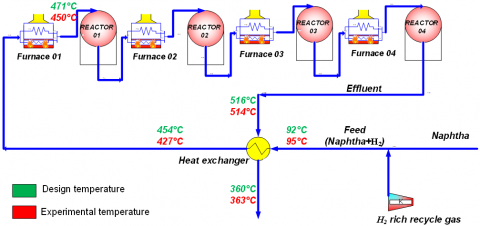Figure 1. Simple flowchart for the case study

3. Industrial Heat Exchanger of RA1K

The catalytic naphtha reforming unit of the SKIKDA refinery uses a stainless steel shell and tube heat exchanger, the geometric parameters are summarized in Table 1. The feed (liquid naphtha and hydrogen rich recycle gas) goes through the shell-side, whereas effluents from flows reactor 4 on the shell-side (Figure 2), this process is a counter-current heat-transfer process. Given that the main target of the estimation is the outlet temperature, plant data (inlet temperatures and mass flow rates) were used for both parameter estimation and a check of simulations. The sharp drop in shell-side flow rates could possibly be responsible for this large decrease in the temperature. In the present study, shell and tube heat exchanger is used to study the various parameters. Data sets utilized for developing this process model were obtained during normal operation.

## 2.png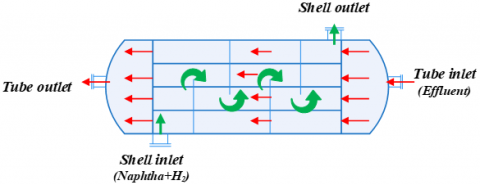Figure 2. Diagram of a typical shell and tube heat exchanger

Table 1. Shell and tube heat exchanger geometry

 Variables Dimension Number of tubes (NT) 1039 Length of tube (LT) 7000 mm Number of shell passes (NP) 1 Number of tube passes (np) 1 Tube outside diameter (d0) 25.4 mm Tube inside diameter (di) 21.184 mm Shell diameter (Ds) 1375 mm Pitch (P) 32.5 mm Distance between baffles (b) 615 mm Tube bundle geometry Triangular
4. Methodology

In this research work, Kern method has been used to check shell and tube heat exchanger in the design and experimental cases [19-21]. Calculations on the side of the tube and the shell have been performed to determine heat transfer coefficient, Overall heat transfer coefficient, overall thermal conductance etc. [22-24]. The mathematical formulas used for the calculations will be presented later on in this article. Then, the Aspen HYSYS software has been used to study the influence of various naphtha mass flow rates and the H2/HC ratio on the thermal performance of a shell and tube heat exchanger.

5. Heat Exchanger Analysis

5.1 Energy balance

According to the first principle of thermodynamics, the heat transfer rate (Q) must also equal the rate of heat lost by the hot fluid stream and gained by the cold fluid stream, the energy balance equations for shell-and-tube heat exchangers are presented below :

Qh = Qc (Energy Balance Equation)

$m_h C_{p, h}\left(T_{h, \text { in }}-T_{h, \text { out }}\right)=m_c C_{p, c}\left(T_{c, \text { out }}-T_{c, \text { in }}\right)$               (1)

where, Tin and Tout are the temperatures of the fluid at the inlet and the outlet respectively.

5.2 Heat transfer rate

5.2.1 Tube side

## 3.png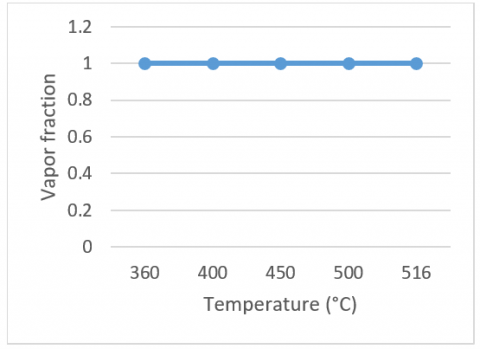Figure 3. The vapor fraction of the heat exchanger inside tubes plotted as a function of the temperature

The vapor fraction of the heat exchanger inside tubes plotted as a function of the temperature is shown in Figure 3. Tube side heat exchanger keeps the fluid (effluent) phase constant throughout the process, steam enters and steam leaves. The rules for single-phase flow are then applied. The heat transfer rate of effluents is calculated as:

$Q_h=m_h C_{p, h}\left(T_{h, \text { in }}-T_{h, \text { out }}\right)$          (2)

5.2.2 Shell side

In shell-side the heat exchanger, two phases are present: a gas phase containing hydrogen and a liquid phase containing naphtha. The vapor fraction of the heat exchanger inside shell plotted as a function of the temperature is shown in Figure 4. As more heat is added the Naphtha progressively changes phase from liquid to vapor while maintaining the temperature at Tv =149℃ (design case).

## 4.png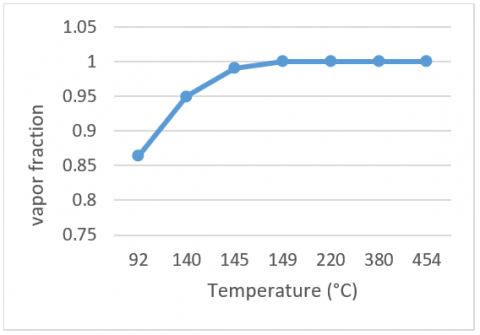Figure 4. The vapor fraction of the heat exchanger inside shell plotted as a function of the temperature

The rules for two-phase flow are then applied. The heat transfer rate of feed is calculated as :

$Q_c=\left[\left(m_v C_{p, v}\right)+\left(m_l C_{p, l}\right)\right]\left(T_v-T_{c, \text { in }}\right)+L_v m_l+m_T C_{p, T}\left(T_{c, \text { out }}-T_v\right)$       (3)

5.3 Heat transfer coefficient

5.3.1 Tube side heat transfer coefficient

The tube side heat transfer coefficient (hi) can be determined as follows :

$\mathrm{h}_{\mathrm{i}}=\mathrm{j}_{\mathrm{h}} \frac{\lambda_{\mathrm{i}}}{\mathrm{d}_{\mathrm{i}}}\left(\frac{\mathrm{C}_{\mathrm{pi}} \mu_{\mathrm{i}}}{\lambda_{\mathrm{i}}}\right)^{\frac{1}{3}}$          (4)

λi is the thermal conductivity, Cpi is the speciﬁc heat, μi is the viscosity of the fluid in tube side at wall temperature, its value is not known a priori but it is calculated at (Tm).

The average fluid temperature (Tm) on the tube side was calculated using:

$T_m=\frac{\mathrm{T}_{\mathrm{in}}+\mathrm{T}_{\mathrm{out}}}{2}$         (5)

where, jh is dimensionless thermal factor according to Kern method can be obtained from Figure A1 in Appendix.

Re is the Reynolds number in tube side, defined as in Eq. (6). It represents the vapor phase only flowing alone in the complete cross-section of the tube at the total mass velocity.

$\operatorname{Re}=\frac{\mathrm{G}_{\mathrm{t} \mathrm{d}_{\mathrm{i}}}}{\mu}$            (6)

Gt is the mass velocity, also known as mass flux, is defined by the mass flow rate divided by the total cross-sectional area, is calculated as Eq. (7).

$\mathrm{G}_{\mathrm{t}}=\frac{\mathrm{m}_{\mathrm{t}}}{\mathrm{a}_{\mathrm{t}}}$         (7)

at is tube side ﬂow cross sectional area (m2) per tube pass, expressible as:

$a_t=\frac{N t \pi i^2}{n_p 4}$       (8)

5.3.2 Shell side heat transfer coefficient

Many researchers proposed nondimensional correlations for forced convective heat transfer on different heat exchangers. Mandrusiak and Carey ; Wen and Ho ; Qiu and Zhang . The general correlation for boiling heat transfer is given by Eq. (9). The correlation is represented using the Lockhart-Martinelli parameter X. In their research, they defined X as Xtt, where the liquid and vapor flowed turbulently. The subscript tt indicates that both phases are turbulent. Modes of calculating the Lockhart-Martinelli parameter for one of the two fluids moving in the laminar regime (Xlt, Xtl, Xll) have been provided in the literature, but they are not necessary in this study.

McNaught  noted out that the relationships all ignore the interacting effects of vapor shear and inundation, and proposed that shell-side condensation at high vapor velocities be considered as two-phase forced convection. He therefore presumed that the high vapor velocity data can be correlated with Eq. (9).

$\frac{\mathrm{h}_{\mathrm{tp}}}{\mathrm{h}_{\mathrm{l}}}=\mathrm{a}\left(\frac{1}{\mathrm{X}_{\mathrm{tt}}}\right)^{\mathrm{b}}$            (9)

The Martinelli parameter Xtt is defined as :

$X_{t t}=\left(\frac{1-y}{y}\right)^{0.9}\left(\frac{\rho_{\mathrm{v}}}{\rho_1}\right)^{0.5}\left(\frac{\mu_1}{\mu_{\mathrm{v}}}\right)^{0.1}$             (10)

The single-phase heat transfer coefficient for liquid alone can be calculated by using the Dittus-Boelter/McAdams equation [19, 29]:

$\mathrm{h}_1=0.023\left(\frac{\lambda_{\mathrm{l}}}{\mathrm{d}_{\mathrm{e}}}\right)\left(\operatorname{Re}_1\right)^{0.8}\left(\operatorname{Pr}_1\right)^{0.4}$               (11)

The Prandtl number (Pr) is the ratio of the molecular diffusivity of momentum to the molecular diffusivity of heat, which can be expressed as:

$P r_l=\frac{C p_l \mu_l}{\lambda_l}$            (12)

Rel is the Reynolds number in shell side, calculated as in Eq. (13).

$\operatorname{Re}_1=\frac{\mathrm{G}_{\mathrm{s}}(1-\mathrm{y}) \mathrm{D}_{\mathrm{e}}}{\mu_{\mathrm{l}}}$             (13)

Gs is shell side mass velocity, defined by:

$\mathrm{G}_{\mathrm{s}}=\frac{\mathrm{m}_{\mathrm{s}}}{\mathrm{a}_{\mathrm{s}}}$            (14)

De is referred as the shell side equivalent diameter. For triangular pitch arrangement it is determined as Eq. (15).

$\mathrm{D}_{\mathrm{e}}=4\left(\frac{\frac{\sqrt{3}}{4} P^2-\frac{\pi}{8} d_o}{\frac{\pi d_o}{2}}\right)=\frac{3,46 p^2}{\pi d_o}-d_0$                  (15)

as is shell side ﬂow cross, defined by:

$\mathrm{a}_{\mathrm{s}}=\frac{\mathrm{D}_{\mathrm{s}}}{\mathrm{p}}\left(\mathrm{p}-\mathrm{d}_0\right) \mathrm{b}$               (16)

5.4 Overall heat transfer area

The surface area (A) of a shell and tube heat exchanger can be expressed as [25, 30]:

$A=\frac{Q}{U L M T D}$      (17)

The LMTD method of heat exchanger analysis is based on using the Eq. (18). LMTD is the log mean temperature difference and described as:

$L M T D=\frac{\Delta \mathrm{T}_1-\Delta \mathrm{T}_2}{\operatorname{Ln} \frac{\Delta T_1}{\Delta T_2}}$            (18)

For a counter flow arrangement the ∆T’s are therefore ∆T1 = (Th,in-Tc,out) and ∆T2 = (Th,out-Tc,in).

Figure 5 depicts the heat transfer diagram along the heat exchangers. It was assumed that the heat transfer areas in the heat exchanger are divided into two zones (preheating zone and superheating zone) . The heat transfer area of each region is a portion of the total heat transfer area of the heat exchanger, and defined as:

$\mathrm{A}=A_p+A_S$      (19 )

## 5.png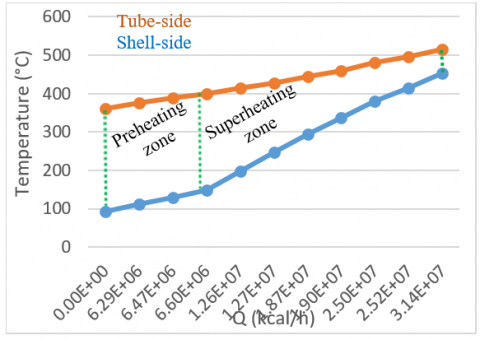Figure 5. Variations of the temperature of the heat exchanger in terms of the heat transfer rate

Preheating zone

The heat transfer area in preheating zone (Ap) is calculated as :

$A_p=\frac{Q_p}{U_p \text { LMTD }_p}$        (20)

The overall heat transfer coefficient (Up) in preheating zone can be expressed by the following Eq. (21):

$\frac{1}{\mathrm{U}_{\mathrm{p}}}=\frac{1}{\mathrm{~h}_{\mathrm{i} 0}}+\frac{1}{\mathrm{~h}_{\mathrm{tp}}}+\sum \mathrm{R}_{\mathrm{f}}$              (21)

where, $\frac{1}{h_{t p}}, \frac{1}{h i o}$ and Rf are tube-and shell-side heat transfer coefﬁcients (h.m2°C/kcal), and tube-and shell-side fouling resistances (h.m2°C/kcal), respectively.

$\mathrm{h}_{\mathrm{i} 0}=\mathrm{h}_{\mathrm{i}} \frac{\mathrm{d}_{\mathrm{i}}}{\mathrm{d}_0}$           (22)

Superheating zone

The heat transfer area in superheating zone (As) is calculated as :

$\mathrm{A}_{\mathrm{s}}=\frac{\mathrm{Q}_{\mathrm{s}}}{\mathrm{U}_{\mathrm{s}} \mathrm{LMTD}_{\mathrm{s}}}$           (23)

The overall heat transfer coefficient (Us) in superheating zone can be expressed by the following Eq. (24):

$\frac{1}{\mathrm{U}_{\mathrm{s}}}=\frac{1}{\mathrm{~h}_{\mathrm{i} 0}}+\frac{1}{\mathrm{~h}_0}+\sum \mathrm{R}_{\mathrm{f}}$            (24)

6. Aspen HYSYS Simulation for Heat Exchanger

Aspen HYSYS software was used to simulate the heat exchanger. The components chosen in Aspen HYSYS were naphtha, recycle gas, and effluent compositions (See Appendix Table A1-A3). The thermodynamic model is Peng Robinson as a fluid package for the simulation basis. On the other hand, for component labelled ‘inlet hot’ was filled with effluent in component selection, and in the component that was labelled ‘Inlet cold’ was filled with Naphtha and recycle gas in component selection. The working conditions include input temperatures, mass ﬂowrate, pressure, and compositions provided from real project executed by RA1K. The design suggested to pumped naphtha and recycle gas in shell side and hot effluent in tube side. The naphtha mass flow is 7.28×104 kg/h (100%), according to the configuration of the design. The heat exchanger was simulated and analyzed under the conditions of 100, 90, 80, 70 and 60 % of naphtha mass flow rate (the corresponding mass flow of naphtha is 7.28×104, 6.55×104, 5.83×104, 5.09×104 and 4.36×104 kg/h, respectively). The other values were left blank and Aspen HYSYS was used to simulate it.

7. Results and Discussion

A study is done on the shell and tube (H2, HC/effluent) heat exchanger and various parameters are calculated for different mass flow rates and at varying inlet and outlet temperatures. Calculations shown in Tables 2 and 3 are made using Kern method for the two cases design and experimental (the corresponding mass flow of naphtha is 7.28×104 and 4.36×104 kg/h, respectively). And further readings are shown for different flow rates and comparison graphs are drawn. The Kern method is used for tube side heat transfer coefficient evaluation; for shell side heat transfer coefficient calculations, the Lockhart-Martinelli correlation is utilized in turbulent flow, the single-phase heat transfer coefficient for liquid alone was obtained using the Dittus-Boelter/McAdams equation.

According to the design, the parameters of the exchanger calculated by the Kern method are as follows: The temperature at the outlet of the shell side exchanger is 456°C, so this temperature is very close to the temperature according to the design (454℃), i.e. the exchanger is able to reach the desired temperature at the outlet of the shell side exchanger. In the experimental case, the Kern method was used to determine the value of the feed temperature at the outlet of the exchanger on the shell side, which is about 430°C. It can be seen that this temperature is low compared to the one expected according to the design (454℃); however, the value of the temperature currently measured in the unit is about 427°C, this temperature (427℃) is very close to the determined temperature (430℃), and although the feed entering the heat exchanger with a temperature of 95℃ instead of 92℃ in the design case (there is therefore a temperature gain of 3℃) it remains that the outlet temperature (427℃) is lower than that of the design 454℃. And this loss of 27℃ in temperature influences the quality of the gasoline since 2℃ increases a RON number.

The computed overall thermal conductance (UA), the total heat transfer rates, the outlet temperature of shell-side heat exchanger, the log-mean temperature difference (LMTD) and H2/HC ratio for two cases (design and experimental) are shown in Table 4. From the results given in Table 4, for the design case, the computed overall thermal conductance by using the Kern method and the HYSYS simulation software were calculated to be 2.09×105 and 2.13×105 kcal/°C.h, respectively, but this computed value was about 1.08×105 and 1.14×105 kcal/℃.h for the experimental case.

Table 2. The results for the shell side are shown below

 Description Design Experimental Tc, in (℃) 92 95 Tc, out (℃) 457 430 mH2 (kg/h) 2.47×104 1.76×104 mnaphtha (kg/h) 7.28×104 4.36×104 CP, H2 (Kcal/kg.℃) 0.7599 0.7690 Cp, ,naohtha (Kcal/kg.℃) 0.5908 0.5889 Cpt (Kcal/kg.℃) 0.8172 0.8650 Lv (Kcal/kg) 79.2 79.0 as (m2) 0.1847 0.1847 De (m) 0.0247 0.0247 Gs (kg/h.m2) 52.83×104 33.21 ×104 Xtt 0.1465 0.1199 Rel 7.15×103 4.016×103 Prl 5.3737 5.37 hl (Kcal/h.m2.℃) 235.03 147.36 htp (Kcal/h.m2.℃) 21.48×102 14.89×102 jh 138 135 h0 (Kcal/h.m2.℃) 747.54 712.87

Table 3. The results for the tube side are shown below

 Description Design Experimental meffluents (kg/h) 16.94×104 10.44×104 Th, out (℃) 360 363 Th, in (℃) 516 514 at (m2) 0.366 0.366 Gt (kg/h.m2) 46.30 ×104 28.54 ×104 Re 65.34×103 40.27×103 jh 165 128 Cpi (Kcal/kg.℃) 1.188 1.189 μi (kg/m.h) 0.1501 0.1501 hi (Kcal/h.m2.℃) 14.52×102 11.27×102 hi0 (Kcal/h.m2.℃) 12.11×102 9.40×102

The comparisons presented by Table 4, show that the agreement between the results obtained by the Kern method and the simulated results is quite good. As the results obtained by the simulation gives temperatures at the outlet of the shell-side heat exchanger close to the Kern method. For this we will carry out a technical study to find a solution. Then, with the HYSYS simulation software, variations of the shell side heat transfer coefficient and overall thermal conductance vs naphtha mass flow are investigated. Finally, the effects of H2/HC ratio and different mass flow of the feed on outlet temperature of shell-side heat exchanger are investigated.

Table 4. Results of analytical and numerical calculations for the design and experimental cases

 Kern HYSYS Simulation Description Design Experimental Design Experimental U.A (Kcal/℃​. h) 2.09×105 1.08×105 2.13×105 1.14×105 Q (Kcal/h) 31419086 18757030 31470085 18783507 Tc, out (℃) 457 430 453 431 LMTD (℃) 146.2 162.5 147.8 164.6 H2/HC 4.68 5.56 4.68 5.56

7.1 Eﬀect of naphtha mass flow on heat transfer coeﬃcient

Figure 6 show the variation of the two phase heat transfer coefficient versus mass flow rate ratio. Also, the mass flow rate ratio (γ) is determined as follows:

$\gamma=\frac{\mathrm{m}_{\mathrm{H} 2}}{\mathrm{~m}_{\mathrm{H} 2}+\mathrm{m}_{\text {naphtha }}}$            (25)

## 6.png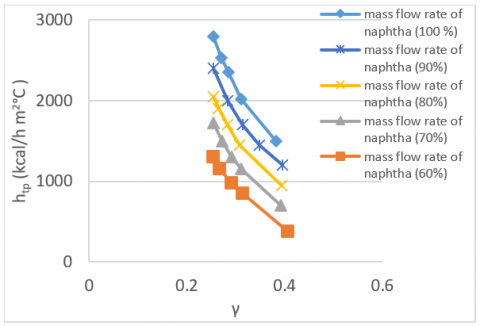Figure 6. Variations of heat transfer coefficient of hydrogen-naphtha the two phase flow vs (γ)

From Figure 6, it is observed that raising the naphtha mass flow rate induces an increase in the two phase heat transfer coefficient. Also, it could be seen that the mass flow rate ratio is an important parameter influencing on the trend of the heat transfer coefficient. By increasing the mass flow rate ratio value, heat transfer coefficient decreases, this phenomenon could be interpreted in a way that as the mass flow rate ratio increases at the constant naphtha mass flow (increment in the hydrogen mass flow rate or decrement in the naphtha mass flow rate), actually, larger amounts of superficial are associated with higher hydrogen flow rates, which would cause bigger slugs. These bigger slugs are less frequent and prevent disruption of the naphtha flow. Bigger slugs could lead in the heat transfer coefficient decreases .

## 7.png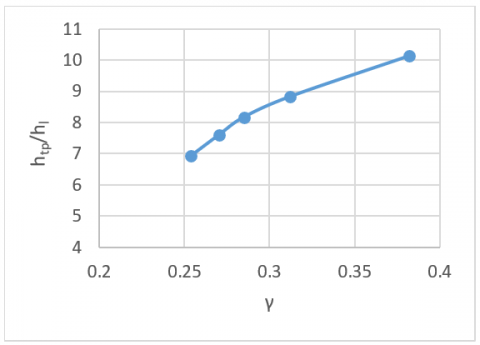Figure 7. Variations of heat transfer coefficient of hydrogen-naphtha two-phase flow per single phase (naphtha) vs (γ)

Figure 7 depicts the relationship between heat transfer ratio (two phase per single phase) and mass flow rate ratio. As shown in Figure 7, the heat transfer coefficient of two phase flow is higher than that of single phase flow. It is believed that at least one of the following mechanisms is responsible for the increase in heat transfer coefficient of two phase flow than that of single phase flow. The mechanisms are as follows:

- The Reynolds number is increasing: according to the continuity equation, injecting of the H2 into the shell reduces the volume fraction of naphtha and as a result the local velocity of naphtha elements increases, as the liquid phase moves quicker, the Re number of the liquid phase also increases. Increment in Reynolds number leads to increment of heat transfer coefficient.

- Interaction between hydrogen bubbles and Naphtha elements: another important mechanism which could responsible for the increase in heat transfer coefficient between the hydrogen bubbles and Naphtha elements. The hydrogen bubbles move along the shell with higher velocity amount than the naphtha elements. However, when hydrogen bubbles move with higher velocity than naphtha elements, the hydrogen bubbles crash with naphtha elements, transmitting the kinetic energy of hydrogen bubbles to naphtha elements making them to have more velocity fluctuations. Subsequently, the turbulence intensity of flow increases, this leads to increment of heat transfer coefficient.

7.2 Eﬀect of naphtha mass flow on overall thermal conductance (UA)

In this paper, overall thermal conductance (UA) is determined by multiplying the overall heat transfer coefficient (U) and the effective surface area (A) of the heat exchanger.

## 8.png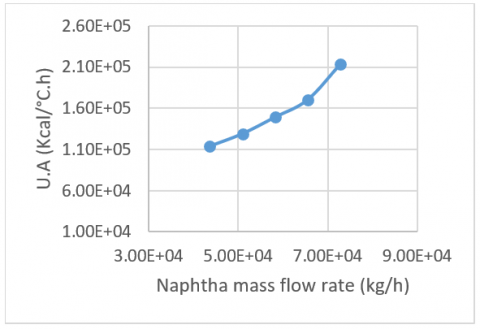Figure 8. Variations of UA in the heat exchanger with the naphtha mass flow rate

## 9.png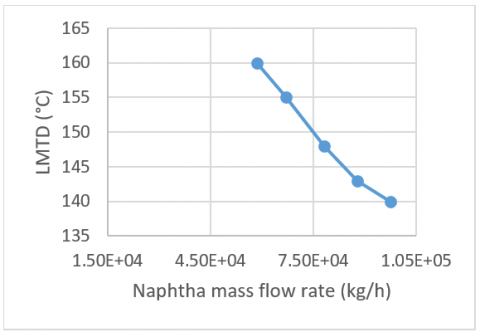Figure 9. Variations of LMTD in the heat exchanger with the naphtha mass flow rate

In the heat exchanger system, the amount of adsorbent mass in the heat exchanger is one of the most influential parameters. Therefore, the influence of naphtha mass flow rate on the system performance varying mass flow rate in shell-side is discussed: Figure 8 shows the effect of mass flow rate in shell-side on overall thermal conductance, where the abscissa represents the mass flow rate of naphtha. In Figure 8, naphtha mass flow rate in shell-side varies from 4.36× 104 to 7.28 ×104 Kg/h. It can be seen from Figure 8 that UA increases with the increase in naphtha mass flow on the shell side. For example, UA is 1.14 × 105 kcal/℃.h at mass flow rate of naphtha of 4.36 × 104 kg/h. With the increase in mass flow rate of naphtha to 5.82 × 104 kg/h, UA increases by 23% to 1.14 × 105 kcal/°C.h. From Figure 8, we can also see that maximum overall thermal conductance (UA) can be obtained at mass flow rate of naphtha about 7.28 × 104 kg/h. Therefore, the change in UA values is mainly due to the change in mass flow rate of naphtha. This is because large UA value means large heat transfer area or high heat transfer coefficient or both, which results in high performance.

Based on Figure 9, it can be seen that with increasing the mass flow rate ratio value the logarithmic temperature difference (LMTD) of the heat exchanger has decreases, this leads to increment of overall heat transfer coefficient of the heat exchanger. The lowest logarithmic temperature difference (LMTD) occurred for mass flow rate of 7.28 ×104 Kg/h. The amount of LMTD was about 143℃.

Figure 10 shows the variations of the overall heat transfer coefficient of the heat exchanger vs of the heat transfer rate. As shown in Figure 10, the total heat transfer coefficient has also increased with increasing fluid heat transfer rate. The highest heat transfer rate occurred for a mass flow of 7.28×104 Kg/h. The amount of heat transfer was about 31470085 kcal/h.

## 10.pngFigure 10. Variations of U in the heat exchanger with the heat transfer rate

7.3 Eﬀect of H2/HC ratio on outlet temperature of shell-side heat exchanger

The hydrogen/hydrocarbon (H2/HC) ratio is defined as the number of moles of hydrogen recycled per mole of naphtha charged to the unit. For studying the influence of the H2/HC ratio on outlet temperature of shell-side heat exchanger, using the HYSYS simulation software, the following procedure can be used: The mass flow rate of the naphtha in the experimental case and the inlet temperatures are fixed and the hydrogen flow rate sent by the compressor is varied. The summary of the results so obtained have been presented in Table 5.

Table 5. Influence of H2/HC ratio on outlet temperature of shell-side heat exchanger

 Mass flow rate of naphtha (kg/h) Mass flow rate of H2 (kg/h) H2 /HC ratio Temperature (Tc, out) (℃) 43687 17656 5.56 428.4 43687 16500 5.19 438.0 43687 16000 5.04 442.3 43687 15500 4.88 446.7 43687 15000 4.72 451.2 43687 14868 4.68 454.0

Figure 11 shows the variations of the outlet temperature of shell-side heat exchanger vs of the H2/HC ratio. As shown in Figure 11, it can be seen that the gradual decrease in the H2/HC ratio makes it possible to increase the outlet temperature of shell-side heat exchanger. The highest outlet temperature of shell-side heat exchanger occurred for H2/HC ratio of 4.68. The value of outlet temperature of shell-side heat exchanger was about 454℃. We notice that with hydrogen mass flow rate of 14868 kg/h, the value of H2/HC ratio was about 4.68. This value is very close to the value of the H2/HC ratio in the design case (Table 4). Furthermore, outlet temperature of shell-side heat exchanger was about 454℃. This value is very close to the desired value in the design case (454℃).

In the design, case the H2/HC ratio used is about 4.68 (Table 4). But, in the experimental case this ratio is about 5.56 (table 4). Note that the H2/HC ratio in the experimental case is higher than the H2/HC ratio in the design case. That is to say that the quantity of hydrogen put in circulation by the compressor in the experimental case does not correspond to the quantity of hydrocarbon used with a mass flow of 60% of the naphtha where 43687 kg/h.

In the primary reforming reactor, the dehydrogenation of naphthenes is rapid and highly endothermic, a significant temperature decrease happens. This effect is minimized by lowering the hydrogen supply to the first reactor, which also lowers the amount of gas produced as a result of hydrocracking. Under project conditions, in order to protect the catalyst, the H2/HC ratio must be held at or above the minimum value of 3 on the 1st reactor of the magnaforming section, so this value (4.68) is valid for the project conditions.

7.4 Effect of different mass flow of the feed on outlet temperature of the shell-side heat exchanger

In practice, the catalytic reforming unit is currently operating at a naphtha flow rate of 60% of the design flow rate. The hydrogen flow rate used in this case has caused major problems, especially in the outlet temperature of the shell-side heat exchanger. For confirm the results given by the previous study, influence of the H2/HC ratio on the outlet temperature of the shell-side heat exchanger, it was considered to vary this ratio with different mass flow rates of naphtha, according to the following cases: 100%, 90%, 80%, 70% and 60%. Thus, the quantity of naphtha is fixed and the quantity of H2 is varied. The simulation results are shown in Figure 12. As shown in Figure 12, it can be seen that the decrease of the naphtha flow rate does not influence the temperature at the exchanger outlet, but with a hydrogen flow rate corresponding to the naphtha flow rate.

By examining these values, we deduce that it is indeed the H2/HC ratio close to 4.6 that gives the temperature expected according to the design (Table 6).

## 11.png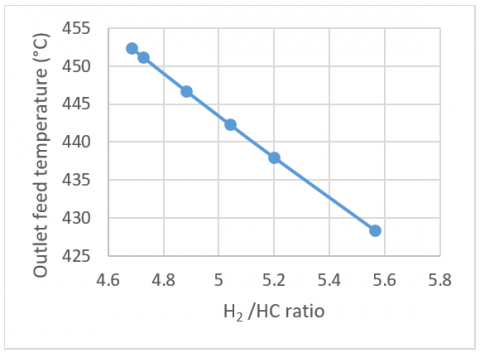Figure 11. Variations of outlet temperature of the shell-side heat exchanger with the H2/HC ratio

## 12.png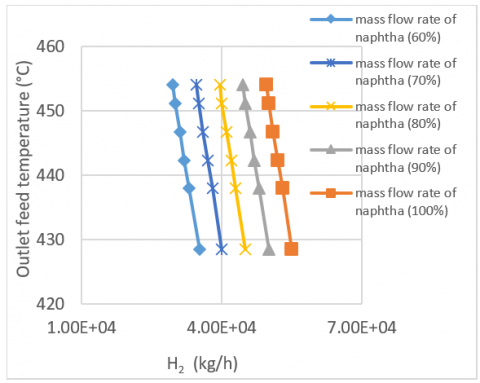Figure 12. Variations of outlet temperature of the shell-side heat exchanger with the H2 mass flow rate

Table 6. Influence of different mass flow of the naphtha on outlet temperature of the shell-side heat exchanger

 Mass flow rate (%) Mass flow rate of naphtha (kg/h) Mass flow rate of H2 (kg/h) H2 /HC ratio Temperature (Tc, out) (℃) 100% 72812 24781 4.68 454 90% 65531 22303 4.68 454 80% 58249 19825 4.68 454 70% 50968 17346 4.68 454 60% 43687 14868 4.68 454
8. Conclusions

In this work the existing heat exchanger of the catalytic naphtha reforming unit was analysed by applying Kern method and Aspen HYSYS simulation software. Experiments were performed to characterize the thermal performances of the heat exchangers. The thermal performance indicators such as the heat transfer coefficient (hi), overall heat transfer coefficient (U), overall thermal conductance (UA), logarithmic temperature difference (LMTD), and mass flow rates for fluids circulating inside the heat exchanger were determined. The results reveal that the ratio two fluids are supplied to the heat exchanger significantly matters in this issue: the flow maldistribution has an important impact on the thermal performances of shell and tube heat exchangers if two fluids are supplied from the same side. Moreover, the flow maldistribution due to due to the decrease in the temperature of the fluids at the outlet of the heat exchanger. It is observed that the degradation in performance is severe for low mass flow rates application. In the present case of shell and tube heat exchanger, it was found that the studied heat exchanger is competent for the duty of heat transfer. It means the feed (naphtha and recycle gas) at 92℃ can be heated to 454℃ by this heat exchanger with hot effluent at 530℃. So, the temperature of feed also can be achieved for low naphtha mass flows rates, but with a H2/HC ratio in the scope of demand (4.68). These results demonstrated the potential of using Aspen HYSYS software to simulate industrial-scale heat exchangers with enhanced heat transfer performances. As a result of this, it is recommended that the refinery modifies the H2/HC ratio currently used.

Acknowledgment

The authors gratefully acknowledge RA1K for the data provided, the industrial members of the catalytic naphtha reforming unit for useful discussions, feedback and encouragement.

Nomenclature
 A Heat transfer area, m2 as Shell-side ﬂow cross sectional area, m2 at Tube-side ﬂow cross sectional area, m2 b Distance between baffles, mm Cp Specific heat, kcal /kg ℃ d0 Tube outside diameter, mm di Tube inside diameter, mm Ds Shell diameter, mm De Diameter equivalent, mm Gs Shell side mass velocity, kg/m2 h Gt Tube side mass velocity, kg/m2 h h heat transfer coefficient, kcal/hm2 ℃ jh thermal factor according to Kern method U Overall heat transfer coefficient, kcal/hm2 ℃ LT Length of tube, mm LMTD Log-mean temperature difference, ℃ Lv Latent heat of vaporization, kcal/kg m Mass flow rate of the fluid, kg/h NT Number of tubes NP Number of shell passes np Number of tube passes P Pitch, mm Pr Prandtl number Q Heat transfer rate, kcal/h Re Reynolds number Rf Fouling resistance, hm2℃/kcal ∆T1 Temperature difference at the hot fluid side, ℃ ∆T2 Temperature difference at the cold fluid side, ℃ Tm Mean fluid temperature, ℃ Greek symbols λ thermal conductivity, kcal/hm℃ γ mass flow rate ratio ρ density, kg/m3 µ dynamic viscosity, kg/m.h Subscripts c Cold e Equivalent h hot in Inlet l Liquid out Outlet s Shell t Tube tp two phase v Vapor
Appendix

Table A1. Composition (mol %): effluent

 Component mol % Hydrogen 82.614 Methane 5.4355 Ethane 2.9396 Propane 2.1284 i-Butane 0.7390 n-Butane 1.0967 i-Pentane 0.2034 n-Pentane 1.4631 n-Hexane 0.3809 n-Heptane 0.2968 n-Octane 0.0185 n-Nonane 0.0086 Cyclohexane 0.0185 1,1 methylcyclopentane 0.0.007 Benzene 0.4273 Toluene 0.7885 Ethylbenzene 0.2649 p-Xylene 0.2112 m-Xylene 0.4027 o-Xylene 0.2312 1 methyl 3- ethylbenzene 0.3260

Table A2. Composition (mol %): recycle gas

 Component mol % Hydrogen 83.9 Methane 4.8 Ethane 4.3 Propane 3.7 i-Butane 1.1 n-Butane 1.4 i-Pentane 0.1 n-Pentane 0.4 n-Heptane 0.3

Table A3. Composition (mol %): Naphtha

 Component mol % i-Pentane 1.09 n-Pentane 1.00 n-Hexane 9.73 n-Heptane 21.27 n-Octane 18.48 n-Nonane 7.49 n-Decane 2.28 Cyclopentane 0.19 Cyclohexane 2.6 Methylcyclopenta 2.12 1,1 methylcyclopentane 13.00 1, 1, 2 methylCyclopentane 8.66 1, 1, 3 methylCyc-C6 3.01 1, 2, 3, 4 titra methyl cyclo C6 0.25 Benzene 1.20 Toluene 2.8 Ethylbenzene 0.61 p-Xylene 0.55 m-Xylene 1.47 o-Xylene 0.78 1, 2, 3 methylbenzene 1.42

## a1.png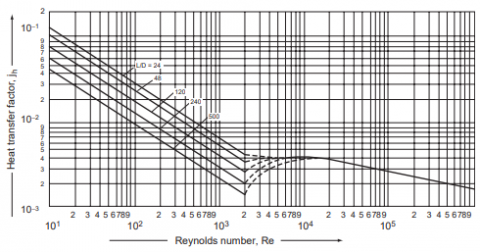Figure A1. Tube side heat transfer factor 

References

 Mehdizadeh-Fard, M., Pourfayaz, F., Mehrpooya, M., Kasaeian, A. (2018). Improving energy efficiency in a complex natural gas refinery using combined pinch and advanced exergy analyses. Applied Thermal Engineering, 137: 341-355. https://doi.org/10.1016/j.applthermaleng.2018.03.054

 Zohuri, B. (2017). Heat exchanger types and classifications. In Compact Heat Exchangers, 19-56. https://doi.org/10.1007/978-3-319-29835-1_2

 Sánchez-Escalona, A., Góngora-Leyva, E. (2019). Improvements to the heat transfer process on a hydrogen sulphide gas coolers system. International Journal of Heat and Technology, 37(1): 249-256. https://doi.org/10.18280/ijht.370130

 De, D., Pal, T., Bandyopadhyay, S. (2017). Helical baffle design in shell and tube type heat exchanger with CFD analysis. International Journal of Heat and Technology, 35(2): 378-383. https://doi.org/10.18280/ijht.350221

 Shweta, Y.K. (2014). Analysis comparing performance of a conventional shell and tube heat exchanger using kern, bell and bell delaware method. International Journal of Research in Engineering and Technology, 3(15): 486-496. https://doi.org/10.15623/ijret.2014.0315093

 Chen, L.Y., Adi, V.S.K., Laxmidewi, R. (2022). Shell and tube heat exchanger flexible design strategy for process operability. Case Studies in Thermal Engineering, 37: 102163-102163. https://doi.org/10.1016/j.csite.2022.102163

 Hu, H.G., Zhang, C. (2007). A modified k-ε turbulence model for the simulation of two-phase flow and heat transfer in condensers. International Journal of Heat and Mass Transfer, 50(9-10): 1641-1648. https://doi.org/10.1016/j.ijheatmasstransfer.2006.10.031

 Lambert, C., Laulan, B., Decloux, M., Romdhana, H., Courtois, F. (2018). Simulation of a sugar beet factory using a chemical engineering software (ProSimPlus ®) to perform Pinch and exergy analysis. Journal of Food Engineering, 225: 1-11. https://doi.org/10.1016/j.jfoodeng.2018.01.004

 Kökdemir, B., Acaralı, N. (2021). A novel study on CHEMCAD simulation of isopropyl alcohol dehydrogenation process development. Journal of the Indian Chemical Society, 98(3): 100035. https://doi.org/10.1016/j.jics.2021.100035

 Hou, W., Su, H., Hu, Y., Chu, J. (2006). Modeling, Simulation and Optimization of a Whole Industrial Catalytic Naphtha Reforming Process on Aspen Plus Platform. Chinese Journal of Chemical Engineering, 14(5): 584-591. https://doi.org/10.1016/S1004-9541(06)60119-5

 Zhang, Y., Wang, D., Yang, J., Tian, L., Wu, L. (2016). Research on the Hydrate Formation in the process of Gas Phase CO2 Pipeline Transportation. International Journal of Heat and Technology, 34(2): 339-344. https://doi.org/10.18280/ijht.340226

 Wang, D., Zhang, Y., Adu, E., Yang, J., Shen, Q., Tian, L., Wu, L. (2016). Influence of Dense Phase CO2 Pipeline Transportation Parameters. International Journal of Heat and Technology, 34(3): 479-484. https://doi.org/10.18280/ijht.340318

 Al-Lagtah, N.M.A., Al-Habsi, S., Onaizi, S.A. (2015). Optimization and performance improvement of Lekhwair natural gas sweetening plant using Aspen HYSYS. Journal of Natural Gas Science and Engineering, 26: 367-381. https://doi.org/10.1016/j.jngse.2015.06.030

 Taqvi, S.A., Tufa, L.D., Muhadizir, S. (2016). Optimization and dynamics of distillation column using aspen plus®. Procedia Engineering, 148: 978-984. https://doi.org/10.1016/j.proeng.2016.06.484

 Janaun, J., Kamin, N.H., Wong, K.H., Tham, H.J., Kong, V.V., Farajpourlar, M. (2016). Design and simulation of heat exchangers using Aspen HYSYS, and Aspen exchanger design and rating for paddy drying application. IOP Conference Series: Earth and Environmental Science, 36: 012056-023056. https://doi.org/10.1088/1755-1315/36/1/012056

 Yandrapu, V.P., Kanidarapu, N.R. (2022). Energy, economic, environment assessment and process safety of methylchloride plant using Aspen HYSYS simulation model. Digital Chemical Engineering, 3: 100019-100019. https://doi.org/10.1016/j.dche.2022.100019

 Yang, M., Li, T., Feng, X., Wang, Y. (2020). A simulation-based targeting method for heat pump placements in heat exchanger networks. Energy, 203: 117907-117907. https://doi.org/10.1016/j.energy.2020.117907

 Rodríguez, M.A., Ancheyta, J. (2011). Detailed description of kinetic and reactor modeling for naphtha catalytic reforming. Fuel, 90(12): 3492-3508. https://doi.org/10.1016/j.fuel.2011.05.022

 Kern, D.Q. (1988). Process Heat Transfer (24. print.). Auckland: McGraw-Hill.

 Towler, G., Sinnott, R. (2021). Chemical engineering design: principles, practice and economics of plant and process design. Butterworth-Heinemann.

 Gaddis, E.S., Gnielinski, V. (1997). Pressure drop on the shell side of shell-and-tube heat exchangers with segmental baffles. Chemical Engineering and Processing: Process Intensification, 36(2): 149-159. https://doi.org/10.1016/S0255-2701(96)04194-3

 Shah, R.K., Sekulic, D.P. (2003). Fundamentals of Heat Exchanger Design. John Wiley & Sons.

 Mujumdar, A.S. (2000). Heat Exchanger Design Handbook T. Kuppan Marcel Dekker Inc., New York 2000, 1118 pages. Drying Technology, 18(9): 2167-2168. https://doi.org/10.1080/07373930008917833

 Mandrusiak, G.D., Carey, V.P. (1989). Convective boiling in vertical channels with different offset strip fin geometries. Journal of Heat Transfer, 111(1): 156-165. https://doi.org/10.1115/1.3250638

 Kakac, S., Liu, H., Pramuanjaroenkij, A. (2002). Heat exchangers: selection, rating, and thermal design. CRC Press. https://doi.org/10.1201/9780429469862

 Wen, M.Y., Ho, C.Y. (2005). Evaporation heat transfer and pressure drop characteristics of R-290 (propane), R-600 (butane), and a mixture of R-290/R-600 in the three-lines serpentine small-tube bank. Applied Thermal Engineering, 25(17-18): 2921-2936. https://doi.org/10.1016/j.applthermaleng.2005.02.013

 Qiu, J., Zhang, H. (2020). Experimental investigation on two-phase frictional pressure drop of R600a and R600a/3GS oil mixture in a smooth horizontal tube. International Journal of Refrigeration, 117: 307-315. https://doi.org/10.1016/j.ijrefrig.2020.04.025

 Marto, P.J. (1984). Heat transfer and two-phase flow during shell-side condensation. Heat Transfer Engineering, 5(1-2): 31-61. https://doi.org/10.1080/01457638408962767

 Mills, A.F. (1999). Heat transfer (2nd ed.). Upper Saddle River, N.J: Prentice Hall.

 Batmaz, E., Sandeep, K.P. (2004). Calculation of overall heat transfer coefficients in a triple tube heat exchanger. Heat and Mass Transfer, 1(1): 1-1. https://doi.org/10.1007/s00231-004-0546-0

 Müller-Menzel, T., Hecht, T. (1995). Plate-fin heat exchanger performance reduction in special two-phase flow conditions. Cryogenics, 35(5): 297-301. https://doi.org/10.1016/0011-2275(95)95347-H